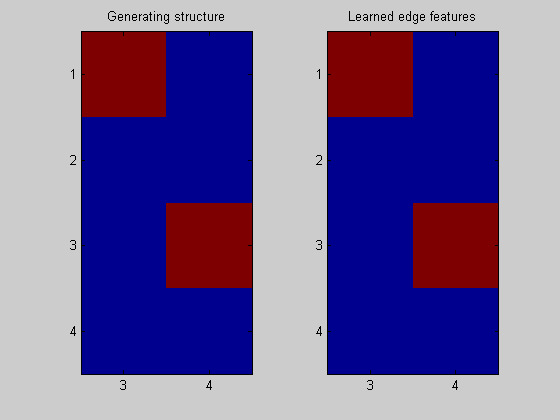## Contents

```% a simple demo of how to do structure learning with data from a DBN
```

## Demo configuration

```rand('seed',1); % Deterministic randomness
randn('seed',1);

HMM = 1;
HUSMEIER = 2;

dbnType = HMM;
```

## Create a DBN

```if dbnType == HMM

% Build a "HMM" model, from which we will sample data
% For the purposes of this demo, the HMM will be fully observed (BNSL cannot handle hidden variables)

% Code that follows is from the BNT documentation
intra = zeros(2);
intra(1,2) = 1; % node 1 in slice t connects to node 2 in slice t

inter = zeros(2);
inter(1,1) = 1; % node 1 in slice t-1 connects to node 1 in slice t

eclass1 = [1 2];
eclass2 = [3 2];
eclass = [eclass1 eclass2];

Q = 2; % num hidden states
O = 2; % num observable symbols

% Original structure: (time flows to the right)
% 2    4
% ^    ^
% 1 -> 3

ns = [Q O];
dnodes = 1:2;
bnet = mk_dbn(intra, inter, ns, 'discrete', dnodes, 'eclass1', eclass1, 'eclass2', eclass2);
prior0 = normalise(rand(Q,1));
transmat0 = mk_stochastic(rand(Q,Q));
obsmat0 = mk_stochastic(rand(Q,O));
bnet.CPD{1} = tabular_CPD(bnet, 1, prior0);
bnet.CPD{2} = tabular_CPD(bnet, 2, obsmat0);
bnet.CPD{3} = tabular_CPD(bnet, 3, transmat0);

maxFanIn = 3; % no fan-in constraint (nNodes-1)

nData = 1000; % sample this many data

% If the distribution is faithful, we should be able to learn (with an infinite sample size):
% 2    4
%      ^
% 1 -> 3
%
% This is exactly what we hoped to learn (when represented as slices we only learn interior edges for the current
% slice (ie. variables 3 & 4) and exterior edges from the past slice to the current slice).
%
% The edges are oriented by the layering (time) constraint.

end
```

## Sample data from the DBN

```data = cell2mat(sample_dbn(bnet, 'length', nData )); % sample some data from the DBN
```

## Convert the data representation to one suitable for direct input into the BNSL routines

Transform the sampled data into a format suitable for BNSL (from time-series to time-slices) Returns a struct holding the new (doubled) # of nodes, a layering that encodes the flow of time, and the transformed data.

```dataDbn = transformDbnData(data, 'maxFanIn', maxFanIn); % *** Important function
```

## Learn the DBN back with the DP algorithm

Since all the parameters are randomly sampled, they could lead to a non-faithful distrubtion (ie. one that encodes conditionally independences not encoded by the DBN).

```% These are the same functions used in simpleDemo.m (they are used throughout the BNSL package)
ep = computeAllEdgeProb( aflp, aflml);

% There is no point in doing structure learning on the first time step, since
% we cannot learn anything from a single data point (in terms of posterior features, such as edges)
% aflp = mkAllFamilyLogPrior( dataDbn.nNodes );
% aflml = mkAllFamilyLogMargLik( data(:,1), 'nodeArity', 2*ones(1,2));
% ep0 = computeAllEdgeProb( aflp, aflml);
```
```Node 1/4
Node 2/4
Node 3/4
Node 4/4
```

## Plot the result

```nNodes = length(bnet.intra);
figure;
subplot(1,2,1); imagesc(bnet.dag(:,nNodes+1:end)); title('Generating structure'); axis('equal'); axis('tight');